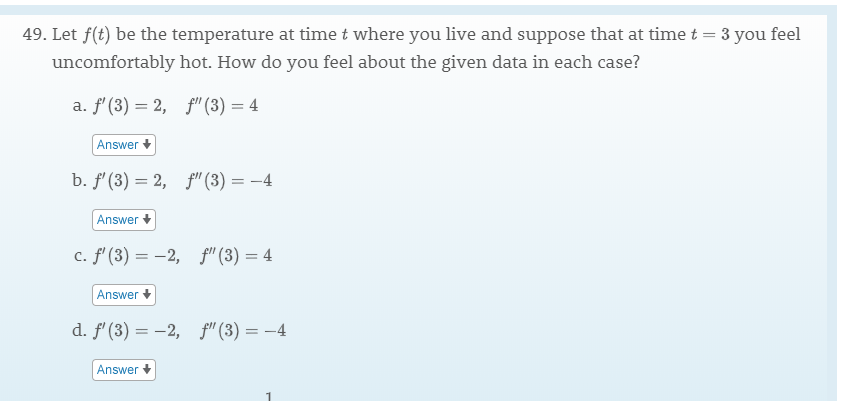# 49. Let f(t) be the temperature at time t where you live and suppose that at time t3 you feeluncomfortably hot. How do you feel about the given data in each case?a. f (3) 2,f"(3) 4Answerb. f (3) 2,f"(3)= -4Answerc. f' (3)2,f" (3) 4Answerd. f (3)2,f (3)4Answer

Question
221 viewshelp_outlineImage Transcriptionclose49. Let f(t) be the temperature at time t where you live and suppose that at time t 3 you feel uncomfortably hot. How do you feel about the given data in each case? a. f (3) 2,f"(3) 4 Answer b. f (3) 2, f"(3)= -4 Answer c. f' (3)2, f" (3) 4 Answer d. f (3)2, f (3)4 Answer fullscreen
check_circle

Step 1

a) f'(3)=2, f"(3)=4

f'(3) > 0 , so the temperature f(t) is  increasing at x=3

f"(3)>0 implies at x=3, f(x) is concave up so rate of change is increasing.

Answer(a): f(x) is increasing and concave up at x=3

Step 2

b) f'(3)=2, f"(3)= -4

f'(3) > 0 , so the temperature f(t) is  increasing at x=3.

f"(3)<0 implies at x=3, f(x) is concave down so rate of change is decreasing.

Answer(b): f(x) is increasing and concave down at x=3

Step 3

c) f'(3)= -2, f"(3)= 4

f'(3) < 0 , so the temperature f(t) is  decreasing at x=3.

f"(3)>0 implies at x=3, f(x) is concave...

### Want to see the full answer?

See Solution

#### Want to see this answer and more?

Solutions are written by subject experts who are available 24/7. Questions are typically answered within 1 hour.*

See Solution
*Response times may vary by subject and question.
Tagged in
MathCalculus

### Other